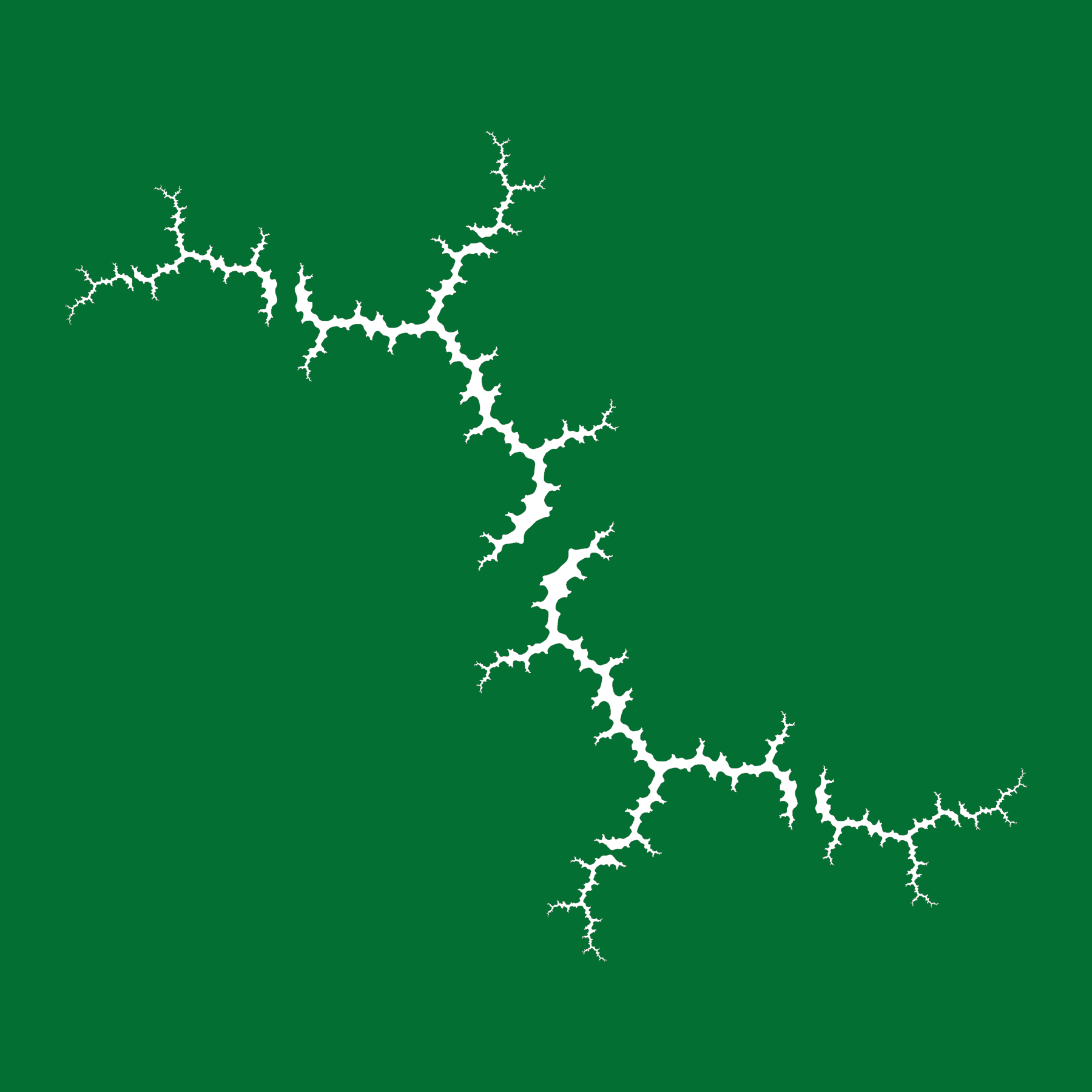Julia Digital Print 2019

The cover art was inspired by the article in this issue by Barnes and Schaubroeck. It shows the filled Julia set of the function $$f_c(z) = z^2 + c$$. Here the value of $$c = (1,92^\circ)$$, expressed using polar coordinates, is based on the number, 1, and volume, 92, of this issue.